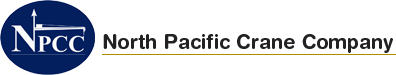### Design Optimization

### Fixed Boom Analysis – for maximun tipping moment prev | next

#### FIXED BOOM ANALYSIS – FOR MAXIMUM TIPPING MOMENT

##### 1 TON Crane
• Stress Plot :
• Max. Working Stress = 17,044 PSI
• Safety factor :
• Min. Safety Factor Plot = 2.1
• DEFORMATION PLOT :
• Max. Deformation = 0.014 INCH

## MCF 1 Ton Stress Plot

##### 2 TON Crane
• Stress Plot :
• Max. Working Stress = 28,245 PSI
• Safety factor :
• Min. Safety Factor = 1.98
• DEFORMATION PLOT :
• Max. Deformation = 0.71 INCH
##### 10 TON Crane
• Stress Plot :
• Max. Working Stress = 28,245 PSI
• Safety factor :
• Min. Safety Factor = 2.5
• DEFORMATION PLOT :
• Max. Deformation = 1.3 INCH

## MCF 1065 Deformation Plot

##### 20 TON Crane
• Stress Plot :
• Max. Working Stress = 28,245 PSI
• Safety factor :
• Min. Safety Factor = 2.5
• DEFORMATION PLOT :
• Max. Deformation = 1.3 INCH